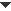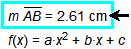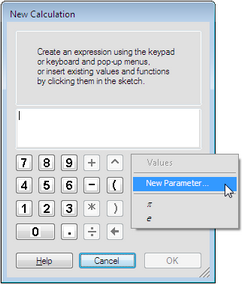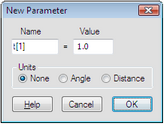﻿ Windows > Calculator

# CalculatorUse the Calculator to create or edit two kinds of Sketchpad objects: calculations and functions.

Calculations: Calculations are values generated using numbers, mathematical operations, functions, measurements, or parameters from your sketch. These calculations can be used for a wide variety of purposes: to define distances, angles, and scale factors by which objects are transformed; to plot points; and to determine the results of other calculations and functions.

Functions: When you define a function in Sketchpad, you can also use numbers, mathematical operations, measurements, parameters, or other functions from your sketch. Once you’ve defined a function, use it for various purposes: plot it, evaluate it, differentiate it, or use it to define other calculations or functions.

There are several different purposes for which you’ll use the Calculator:

To create a new calculation, choose

To create a new function, choose

Choose Graph | Plot New Function to create a new function and plot it immediately.

To edit a calculation, double-click the calculation with the Selection Arrow tool, or select the calculation and choose

To edit a function definition, double-click the function with the Selection Arrow tool, or select the function and choose

To change a parameter into a calculation, select the parameter, choose and change the expression to something more complicated than just a number.

To change a calculation into a parameter, edit it so that it consists of one number only, with or without distance or angle units.Insert a Value or Function from the SketchWhile you’re using the Calculator to define a calculation or a function, you can click existing values or functions in the sketch to insert these objects into your new expression. For instance, if you have a sketch in which you’ve already measured the length of segment AB, you can insert this length into the Calculator’s expression by clicking on the existing measurement in the sketch. Similarly, if you have a sketch in which you’ve defined a function f(x) = ax2 + bx + c, use this function in other calculations and functions by clicking it in the sketch. You can also click a Hot Text link to a value or function to insert that value or function into the Calculator. If a value or function you want to insert is hidden behind the Calculator itself, drag the Calculator to the side so you can click on the object you want to insert.Insert a New ParameterWhile you’re using the Calculator to define a calculation or a function, you can create a new parameter and insert it into your expression. 1.Choose New Parameter from the Values pop-up menu, or use the keyboard shortcut Shift+Ctrl+P (Windows) or Shift-P (Mac). The New Parameter dialog box appears2.Type the name you want to use for the new parameter. You can also set the initial value of the parameter.3.Click OK. The parameter is inserted into your expression and added to your sketch.The result is the same as if you had used Number | New Parameter to create the parameter before using the Calculator.